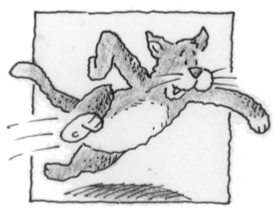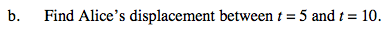### Home > CALC > Chapter 6 > Lesson 6.5.1 > Problem6-172

6-172.
1.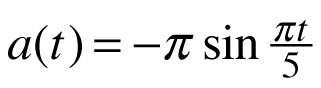Bob's cat, Alice, is still doing wind sprints up and down his hallway. This time suppose that Alice's acceleration after t seconds is given by the formula. As Alice hits the hallway, her velocity is already 6 feet per second, so v(0) = 6. Homework Help ✎

1. Find a formula for Alice's velocity at time t.

2. Find Alice's displacement between t = 5 and t = 10.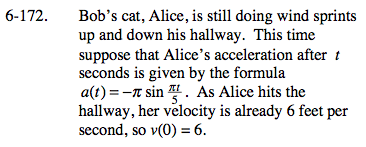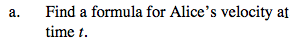To find the velocity formula, will need to use the given initial velocity.